# Can there be negative frequencies and wavelengths

### Bohr's atomic model

If we let white light from the sun or a light bulb pass through a prism, it is then broken down into a wide, uninterrupted ribbon of color. The colors appear in the order of their wavelengths or frequencies. Solids and liquids, some gases under very high pressure (e.g. in the stars) can be made to glow with a large amount of heat. Such light provides one continuous emission spectrum. According to the wave model of light, it is the visible range from the Electromagnetic wave spectrum.

What do the emission spectra of the substances look like in the gaseous atomic state under normal pressure?

First, we vaporize salt solutions of different alkali metals one after the other in the flame of a Bunsen burner. We observe the flame in the dark with a spectral apparatus. A saline solution (NaCl) colors the flame intensely orange, in the spectral apparatus we see a yellow line with a wavelength of approx. 590 nm. Salts of lithium and potassium emit reddish light. The spectrum of lithium are two red lines. The potassium has red and one purple lines in the spectrum, the red lines not matching the spectrum of lithium.

To use a gas, e.g. B. To make hydrogen glow, we ionize it in a glass tube by applying a voltage. A diffraction grating splits the image from our spectral tube into a series of colored parallel images: the visible Emission spectrum of hydrogen: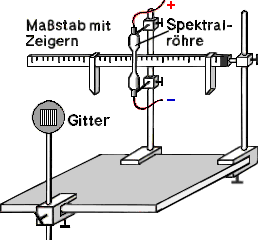By supplying energy, atoms can be made to glow. They only emit light of characteristic wavelengths. Their discrete emission spectrum is therefore called the line spectrum. It is unique for each chemical element. With the spectral analysis chemical elements can be identified.Let us now consider the reverse process: the Absorption of light. To do this, we take a sodium and a mercury vapor lamp and project their light onto a wall. We put a bunsen burner between the wall and the lamps. Its flame is translucent to both light sources. Now we bring a sodium salt solution into the flame. The Na lamp now casts a shadow of the flame on the wall, while the image of the Hg lamp remains unchanged. The Na flame has absorbed the light from the Na lamp, but not the light from the Hg lamp, although mercury also has a yellow line (578 nm) close to the line from the Na spectrum (590 nm) in its emission spectrum.

Atoms absorb light of the same wavelengths that they emit themselves when they are lit.

We can explain this result with quantum theory. Atoms can only absorb or release very specific amounts of energy: quanta of the characteristic energy E =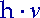. Can an atom with an energyare excited to shine, then exactly the same amount of energy is emitted as a light quantum. Since no line in the Hg spectrum coincides with the Na spectrum, the light from a Hg lamp is made up of other frequencies (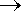other energy quanta) than the light of a Na lamp. This is why the Hg light cannot make Na atoms shine. This quantum mechanical interpretation of the line spectra leads us to Energy level model of the atom:

An atom can only assume states with very specific energies.These energy states are also called energy levels. To transfer the atom from one energy level E. 1 to another energy level E 2 the atom must be exactly the energy quantum according to the law of conservation of energyE = E 2 - E. 1 =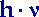pick up or hand over.

1911 proved Ernest Rutherford in scattering experiments the existence of an atomic nucleus with a charge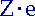, where the Atomic numberZ indicates the number of positive elementary charges e, i.e. protons, in the nucleus. She is the same Atomic number of the element in the periodic table. According to Rutherford, the atomic nucleus is surrounded by a negatively charged shell made of electrons.
Niels Bohr developed this atomic model further. He assumed that the transitions from one energy level to another are made by electrons. Published in his 1913 Postulates Bohr still considered the electrons to be classical particles:

Bohr's postulates:

1. Electrons can only move on defined circular paths around the atomic nucleus. Any such train (also called bowl)corresponds to a very specific energy value: energy level. Each lane is marked with a letter K, L, M, N, ... or an integer 1, 2, 3, 4,..., the so-called Quantum number n, designated. An electron has the lowest energy in an atom when it is on the innermost orbit (K-Bowl, n = 1) is located.

2. Electrons rotate radiation-free (without loss of energy) and with a certain constant orbital velocity vn at a distance rn around the atomic nucleus. The speed vn is determined from the quantum condition:

3.An electron absorbs or emits energy only when there is a sudden transition from one energy level to another (quantum leap). It must not assume any energy values ​​between two permitted, successive energy levels. In order to move an electron from an inner orbit m to a further outer orbit n, work has to be done against the electrostatic attraction between the positively charged nucleus and the negatively charged electron. Therefore an amount of energy is added to itnecessary, which is the difference between the energy levels En-Em corresponds to. When an electron falls back from orbit n to orbit m, it becomes the same amount of energyin the form of a photon free:E = En-Em =(10.2)

 Based on these postulates, Bohr calculated the radii and energy levels of the Hydrogen atom. A hydrogen atom consists of only one proton, which is orbited by an electron. As the centripetal force Fzthe electrostatic attraction between the proton and the electron, the Coulomb force F, actsel . So we can go into approach Fel = Fz: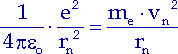(10.3)

for the path speed vn from the Quantum condition insert: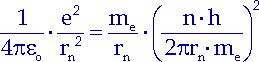(10.4)

and get the Bohr radii:(10.5)

The radius r results for the basic state1 :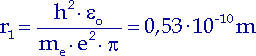(10.6)

This value agrees well with other values ​​found from kinetic gas theory. For each additional electron orbit, according to Bohr: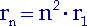(10.7)

The total energy of the electron En is composed of its kinetic and its electrostatic potential energy. The potential energy is negative, because energy has to be applied to remove the electron from the atomic nucleus against the Coulomb force. After solving equation (10.3) for the velocity vn we set the result for vn into the term of kinetic energy. We find that the kinetic energy represents half the amount of the potential energy: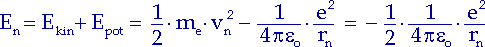(10.8)

We transform this equation with (10.7) and (10.6) and obtain the following relationship: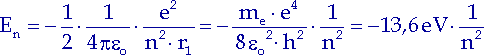(10.9)

An electron in a hydrogen atom can only have the following energy values accept:(10.10)

where the integer ndenotes its current path.

We know from mechanics: In the most stable state, a body has the lowest potential energy. Since the total energy of an electron in an atom is half the potential energy, the most stable state of an atom must be the state of its lowest total energy. He will Basic state called. The hydrogen atom is in the ground state when the quantum number is n = 1. States with larger quantum numbers are less stable, they become excited states called.

Now we can calculate how much energy is consumed or given off when an electron passes from any particular energy level to another particular energy level of the hydrogen atom. This amount of energy only depends on the associated quantum numbers:(10.11)

If an electron is completely separated from the atom, it corresponds to a jump on an infinitely large orbit (m =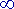). The atom becomes one ion. The one to be expended Ionization energy e.g. from the ground state (n = 1) is 13.6 eV. Conversely, the energy 13.6 eV is released when a proton captures a free, resting electron and forms a hydrogen atom.

Atoms cannot absorb or emit photons of any frequency, but only those whose energy corresponds to the difference between the permitted energy levels:(10.12)

With this formula the spectra of hydrogen and hydrogen-like ions (ions with only one electron in the shell) can be calculated very precisely. The diagram on the right shows us electron transitions from higher energy levels to lower energy levels of a hydrogen atom. Emitted photons of the same frequency appear in the spectrum as a characteristic line. The Emission spectrum of hydrogen besides the visible lines, Balmer series (m = 2, n = 3, 4, 5, ...), also lines in the ultraviolet range, Lyman series (m = 1, n = 2, 3, 4, ...), and in the infrared range, Paschen series (m = 3, n = 4, 5, 6, ...).

However, Bohr's model fails for atoms with more than one electron. Even with helium, the calculated frequencies do not match the spectral lines.
According to Bohr, an electron moves on a circular path, i.e. in a plane, around the atomic nucleus. Kinetic gas theory and experiments showed, however, that hydrogen atoms are not thin disks, but are spherical.
Bohr's quantum condition contradicts the later (1926) established Heisenberg uncertainty principle: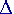px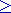h (withp = mev). If the position of the electron (i.e. its orbit and thus the radius) is exactly determined, i. H. the local uncertaintyx is infinitely small, the blurring of its speed becomesvHmex) be infinitely large. According to Bohr, however, the path speed can also be precisely calculated with the radius and vice versa.
The solution to the problem brought wave mechanics from Erwin Schrödinger, in which he treated the electron as a wave with the help of the de Broglie hypothesis.